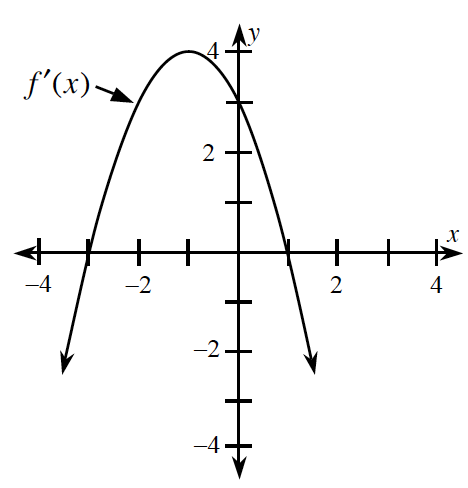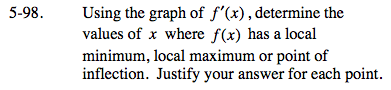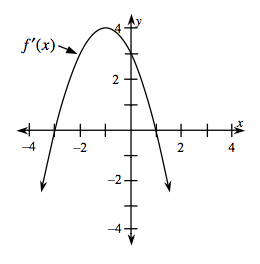### Home > CALC > Chapter 5 > Lesson 5.2.4 > Problem5-98

5-98.
1. Using the graph of f ′(x), determine the values of x where f(x) has a local minimum, local maximum or point of inflection. Justify your answer for each point. Homework Help ✎You are looking at a graph of f '(x), which shows all the slopes of f(x).

A local minimum on f(x) is located where the slopes of f(x) change from negative to positive.A local maximum on f(x) is located where the slopes of f(x) change from positive to negative.

An inflection point on f(x) is where concavity changes, and concavity is a determined by the slope of the the slopes.

x = −3: local minimum
x = 1: local maximum
x = −1: inflection point